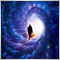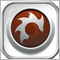# MA of RSI and RSI Indicators in same window734

Hello Forum, good day.

I have the following code that shows the MA of RSI in one window and RSI in another separate window:

```double RSI = iRSI( NULL, 0, RSI_Period, RSI_Price, 1 );

double Get_MA_7_RSI( int shift )
{
double data[];
ArrayResize( data, MA_RSI_Total );

for ( int count = MA_RSI_Total - 1; count >= 0; count-- )
{
data[count] = iRSI( NULL, 0, MA_RSI_Period, RSI_Price, count + shift );
}

return( iMAOnArray( data, MA_RSI_Total, MA_RSI_Period, 0, RSI_MA_Method, 0 ) );
}```

I need them to be displayed in the same window, how can this be done? Your help will be much appreciated.

Best regards and thank you,

codeMolecules1952

Just combine it and make necessary adjustments. One indicator can have many outputs.1466

This is just two RSI, one is smoothed, the other not24283

codeMolecules: I have the following code that shows the MA of RSI in one window and RSI in another separate window:
1. False. Indicator can only write to one window. You have code that shows MA of RSI in one window. PERIOD. The RSI in the other is another indicator.
2. Option one. Change your indicator to display multiple lines.
3. Option two. Drop your code completely and drag the Moving Average on to the RSI window and select source=previous/first indicator.